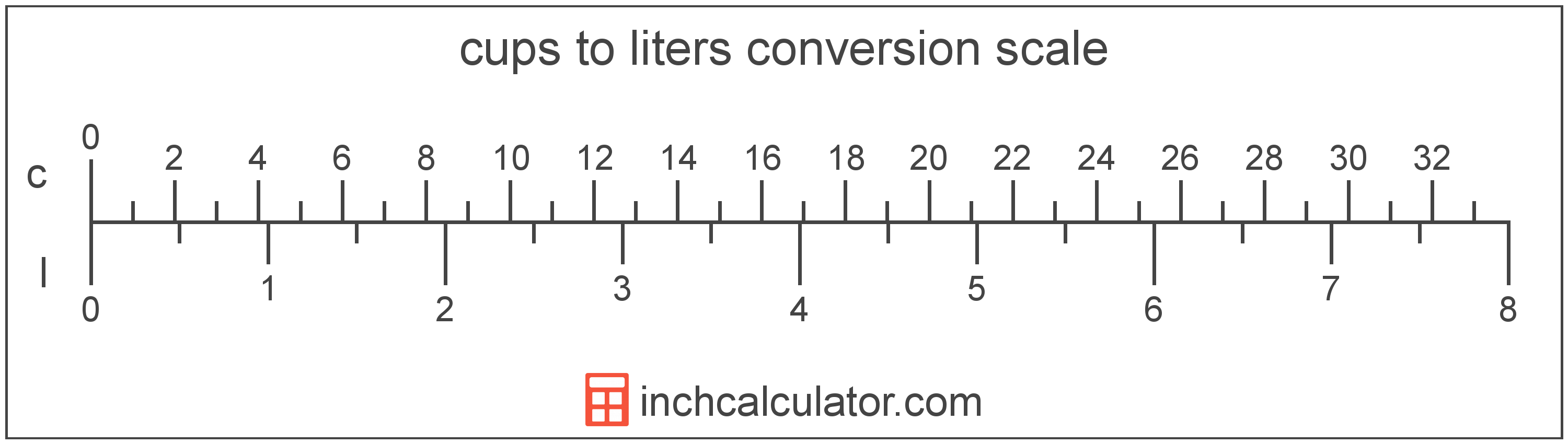Convert Cups to Liters

Enter the volume in cups below to get the value converted to liters.

Results in Liters:1 c = 0.236588 l
Do you want to convert liters to cups?

How to Convert Cups to LitersTo convert a cup measurement to a liter measurement, multiply the volume by the conversion ratio. One cup is equal to 0.236588 liters, so use this simple formula to convert:

liters = cups × 0.236588

The volume in liters is equal to the cups multiplied by 0.236588.

For example, here's how to convert 5 cups to liters using the formula above.
5 c = (5 × 0.236588) = 1.182941 l

Cups and liters are both units used to measure volume. Keep reading to learn more about each unit of measure.

Cups

The cup is a unit of volume equal to 16 tablespoons or 8 fluid ounces. The cup should not be confused with the metric cup or the teacup, which are different units of volume. One cup is equal to just under 236.6 milliliters, but in nutrition labelling, one cup is equal to 240 milliliters.

The cup is a US customary unit of volume. Cups can be abbreviated as c, and are also sometimes abbreviated as C. For example, 1 cup can be written as 1 c or 1 C.

Liters

The liter is a unit of volume equal to 1,000 cubic centimeters. The liter is a special name defined for the cubic decimeter, and is exactly equal to the volume of one cubic decimeter.

The liter is an SI accepted unit for volume for use with the metric system. A liter is sometimes also referred to as a litre. Liters can be abbreviated as l, and are also sometimes abbreviated as L or . For example, 1 liter can be written as 1 l, 1 L, or 1 ℓ.

Cup Measurements and Equivalent Liter Conversions

Common cup values converted to the equivalent liter value
Cups Liters
1 c 0.236588 l
2 c 0.473176 l
3 c 0.709765 l
4 c 0.946353 l
5 c 1.1829 l
6 c 1.4195 l
7 c 1.6561 l
8 c 1.8927 l
9 c 2.1293 l
10 c 2.3659 l
11 c 2.6025 l
12 c 2.8391 l
13 c 3.0756 l
14 c 3.3122 l
15 c 3.5488 l
16 c 3.7854 l
17 c 4.022 l
18 c 4.2586 l
19 c 4.4952 l
20 c 4.7318 l
21 c 4.9684 l
22 c 5.2049 l
23 c 5.4415 l
24 c 5.6781 l
25 c 5.9147 l
26 c 6.1513 l
27 c 6.3879 l
28 c 6.6245 l
29 c 6.8611 l
30 c 7.0976 l
31 c 7.3342 l
32 c 7.5708 l
33 c 7.8074 l
34 c 8.044 l
35 c 8.2806 l
36 c 8.5172 l
37 c 8.7538 l
38 c 8.9904 l
39 c 9.2269 l
40 c 9.4635 l

More Cup Volume Conversions

US Liquid
Convert to Gallons
1 c is equal to 0.0625 gallons
Convert to Quarts
1 c is equal to 0.25 quarts
Convert to Pints
1 c is equal to 0.5 pints
Convert to Fluid Ounces
1 c is equal to 8 fluid ounces
US Cooking
Convert to Tablespoons
1 c is equal to 16 tablespoons
Convert to Teaspoons
1 c is equal to 48 teaspoons
US Customary Volume
Convert to Cubic Inches
1 c is equal to 14.4375 cubic inches
Convert to Cubic Feet
1 c is equal to 0.008355 cubic feet
Convert to Cubic Yards
1 c is equal to 0.000309 cubic yards
Metric Volume
Convert to Milliliters
1 c is equal to 236.588236 milliliters
Convert to Cubic Centimeters
1 c is equal to 236.588236 cubic centimeters
Convert to Cubic Meters
1 c is equal to 0.000237 cubic meters